Скачать презентацию Some Economics of Information Introductory Microeconomics ES 10001

52604a774a266ddbb889348e687f6c0a.ppt

• Количество слайдов: 43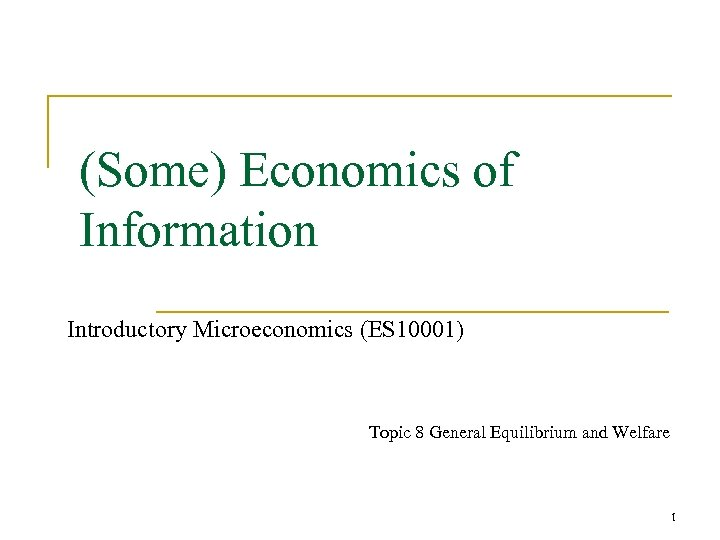(Some) Economics of Information Introductory Microeconomics (ES 10001) Topic 8 General Equilibrium and Welfare 1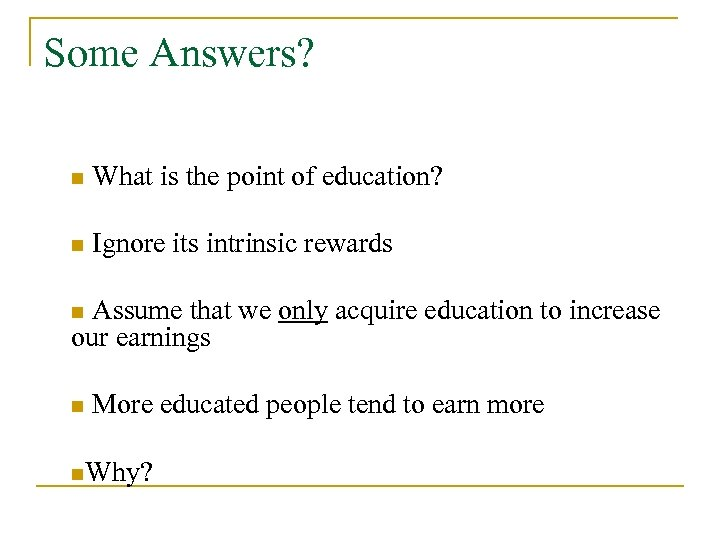Some Answers? n What is the point of education? n Ignore its intrinsic rewards Assume that we only acquire education to increase our earnings n n More educated people tend to earn more n. Why?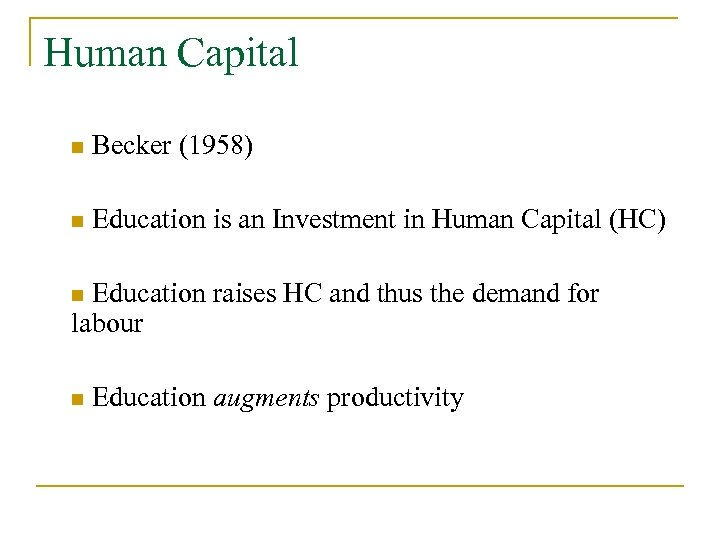Human Capital n Becker (1958) n Education is an Investment in Human Capital (HC) Education raises HC and thus the demand for labour n n Education augments productivity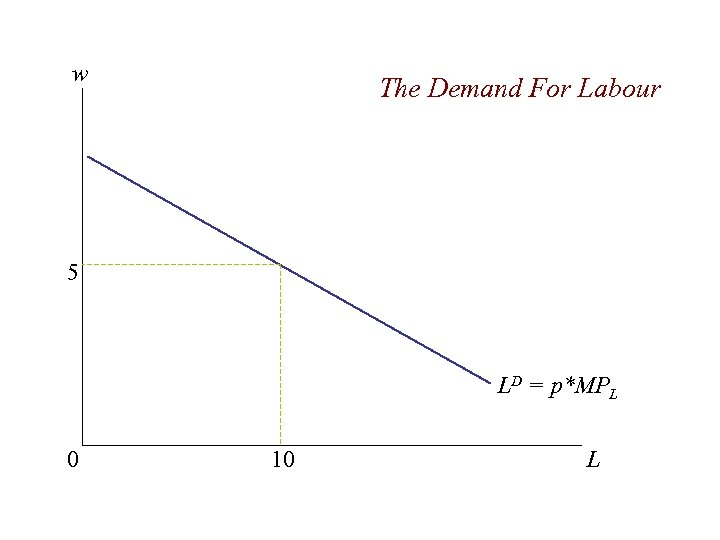w The Demand For Labour 5 LD = p*MPL 0 10 L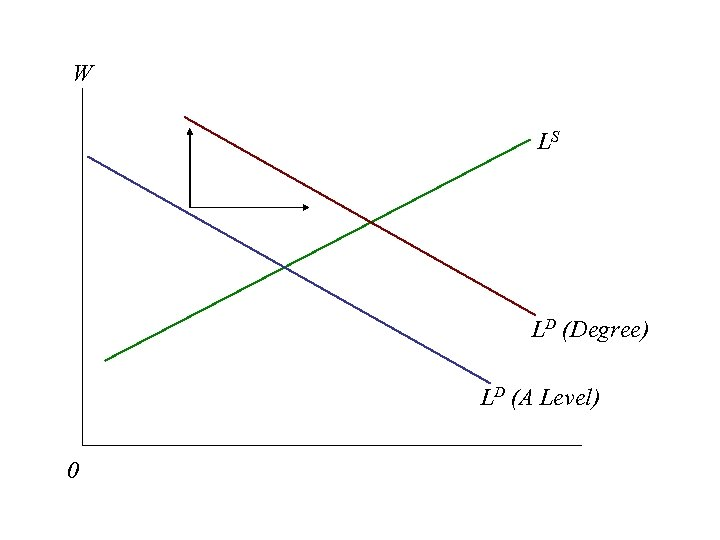W LS LD (Degree) LD (A Level) 0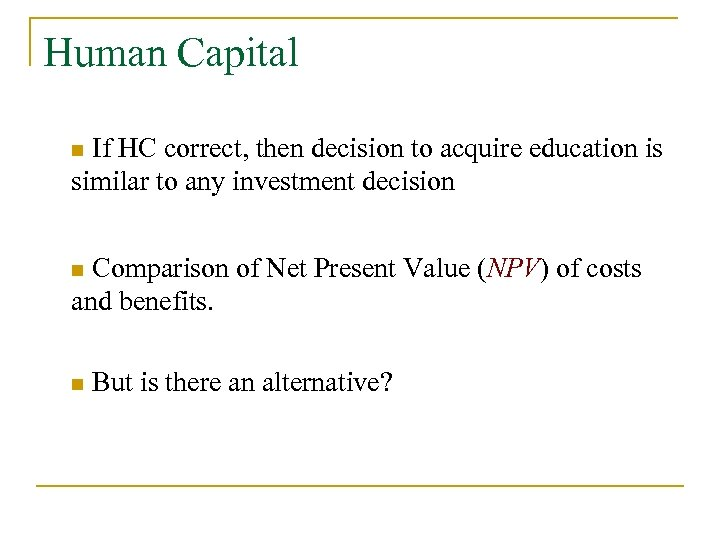Human Capital If HC correct, then decision to acquire education is similar to any investment decision n Comparison of Net Present Value (NPV) of costs and benefits. n n But is there an alternative?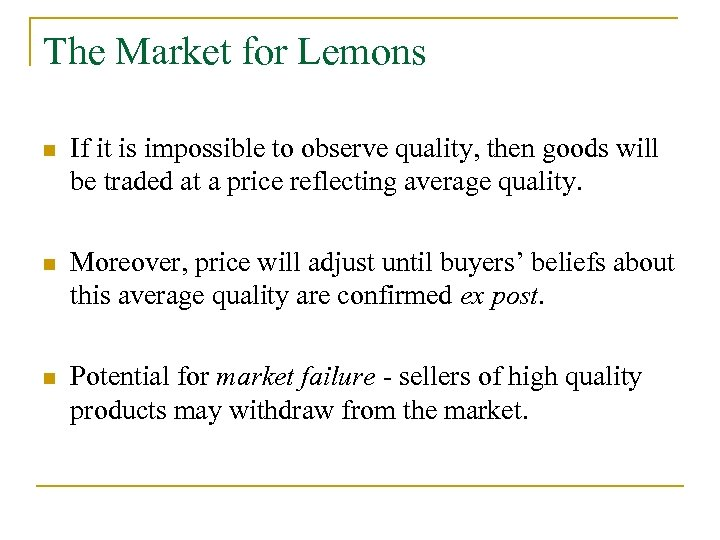The Market for Lemons n If it is impossible to observe quality, then goods will be traded at a price reflecting average quality. n Moreover, price will adjust until buyers’ beliefs about this average quality are confirmed ex post. n Potential for market failure - sellers of high quality products may withdraw from the market.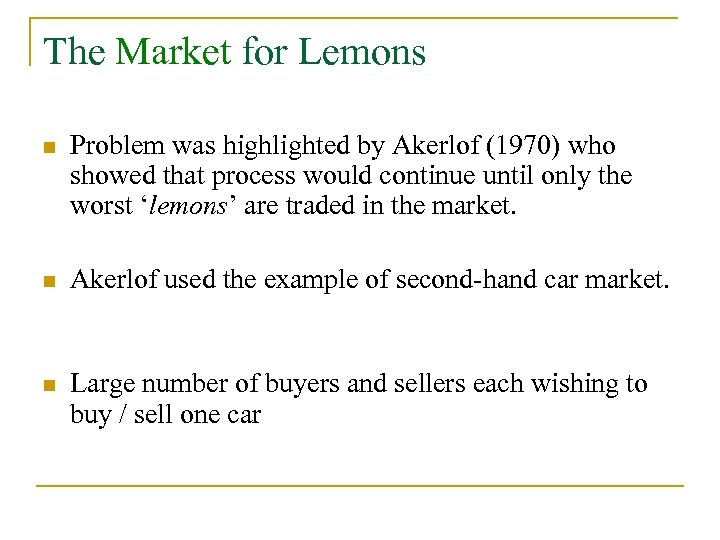The Market for Lemons n Problem was highlighted by Akerlof (1970) who showed that process would continue until only the worst ‘lemons’ are traded in the market. n Akerlof used the example of second-hand car market. n Large number of buyers and sellers each wishing to buy / sell one car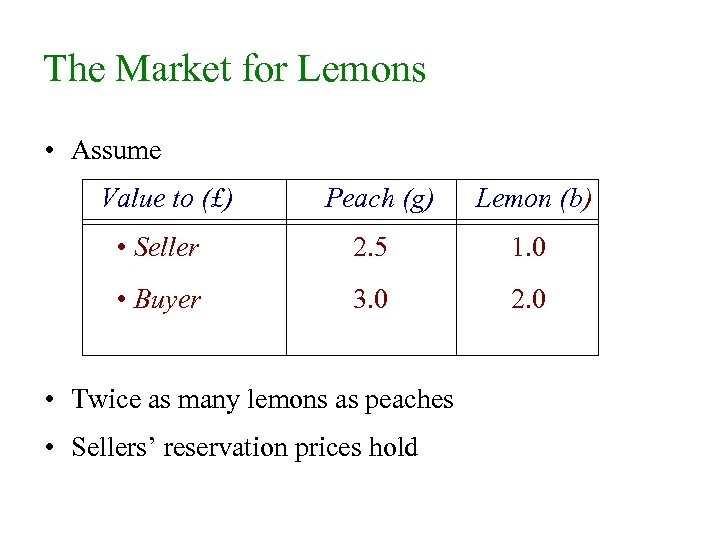The Market for Lemons • Assume Value to (£) Peach (g) Lemon (b) • Seller 2. 5 1. 0 • Buyer 3. 0 2. 0 • Twice as many lemons as peaches • Sellers’ reservation prices hold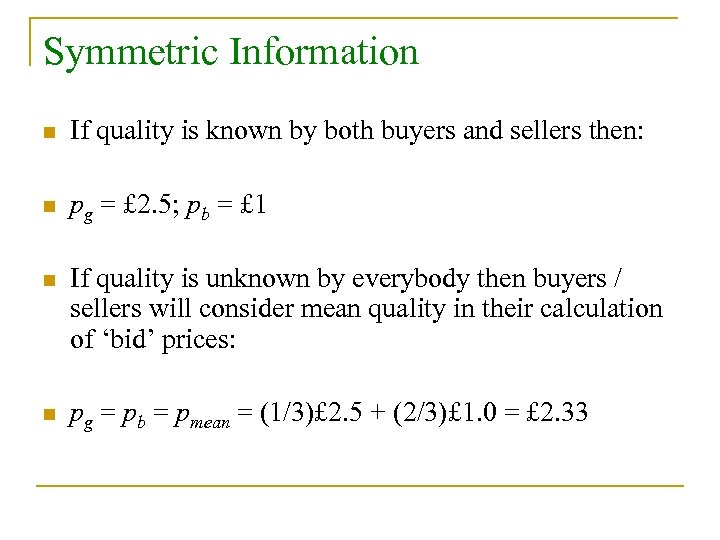Symmetric Information n If quality is known by both buyers and sellers then: n pg = £ 2. 5; pb = £ 1 n If quality is unknown by everybody then buyers / sellers will consider mean quality in their calculation of ‘bid’ prices: n pg = pb = pmean = (1/3)£ 2. 5 + (2/3)£ 1. 0 = £ 2. 33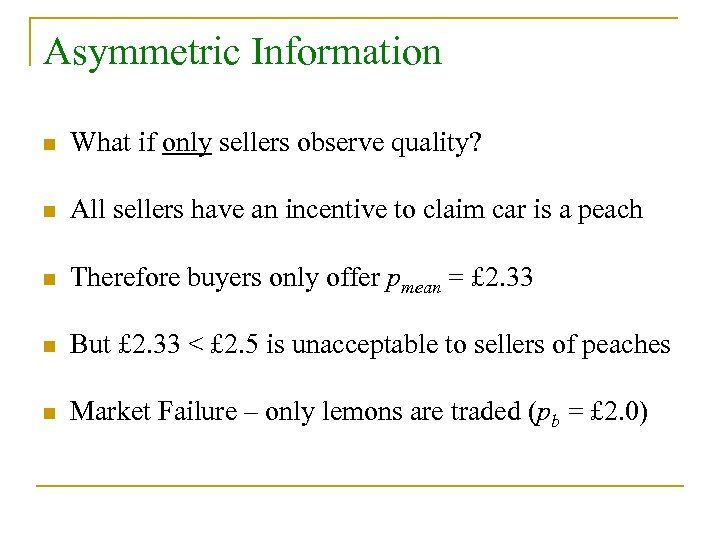Asymmetric Information n What if only sellers observe quality? n All sellers have an incentive to claim car is a peach n Therefore buyers only offer pmean = £ 2. 33 n But £ 2. 33 < £ 2. 5 is unacceptable to sellers of peaches n Market Failure – only lemons are traded (pb = £ 2. 0)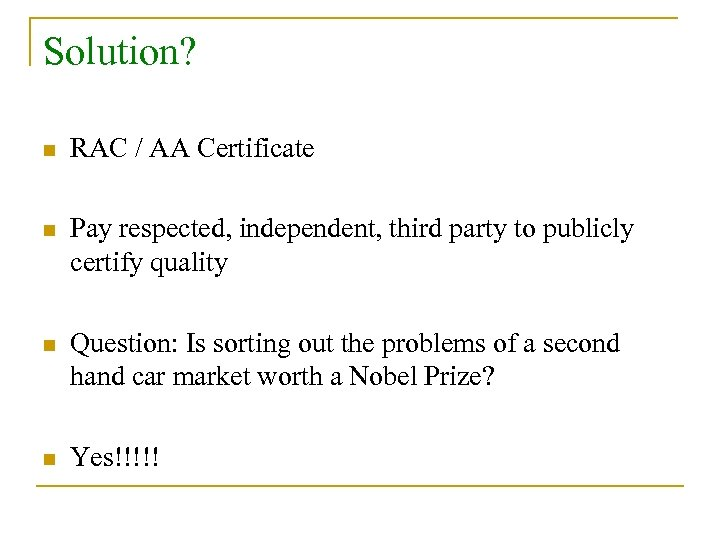Solution? n RAC / AA Certificate n Pay respected, independent, third party to publicly certify quality n Question: Is sorting out the problems of a second hand car market worth a Nobel Prize? n Yes!!!!!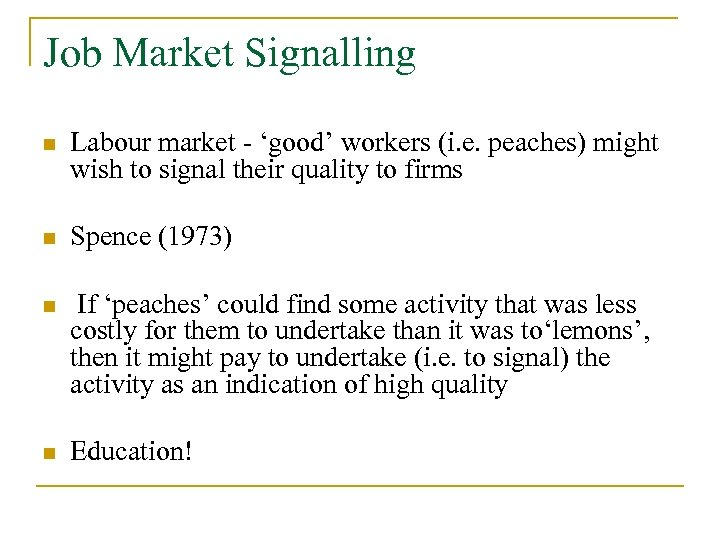Job Market Signalling n Labour market - ‘good’ workers (i. e. peaches) might wish to signal their quality to firms n Spence (1973) n If ‘peaches’ could find some activity that was less costly for them to undertake than it was to‘lemons’, then it might pay to undertake (i. e. to signal) the activity as an indication of high quality n Education!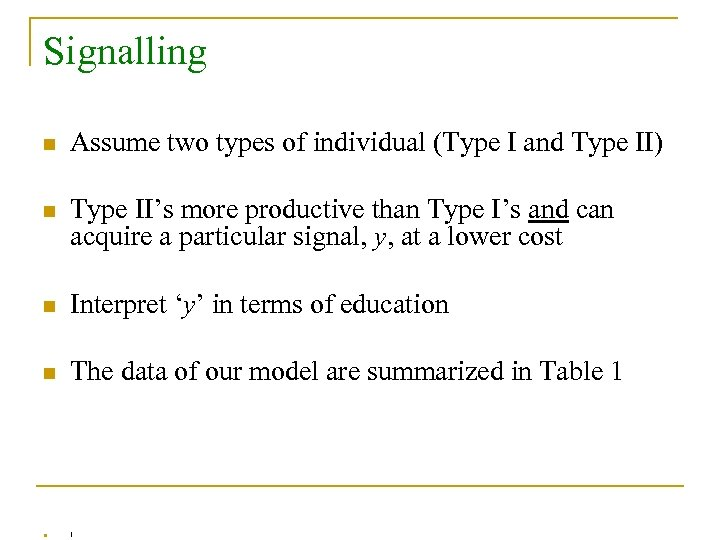Signalling n Assume two types of individual (Type I and Type II) n Type II’s more productive than Type I’s and can acquire a particular signal, y, at a lower cost n Interpret ‘y’ in terms of education n The data of our model are summarized in Table 1 n !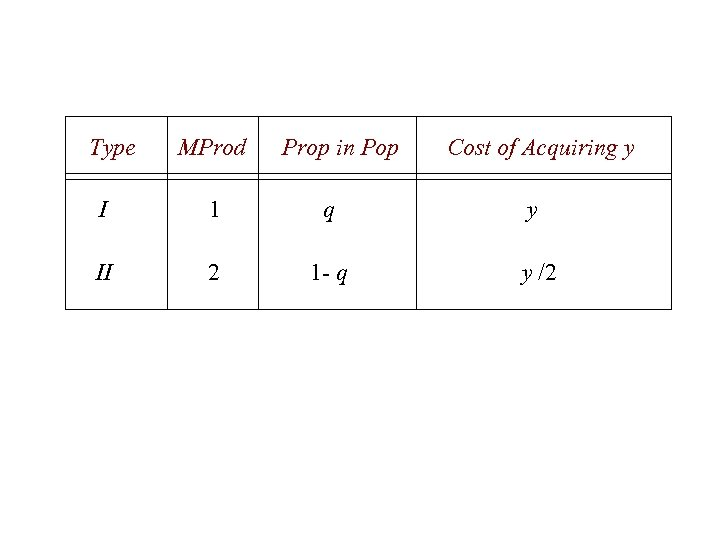Type MProd Prop in Pop Cost of Acquiring y I 1 q y II 2 1 - q y /2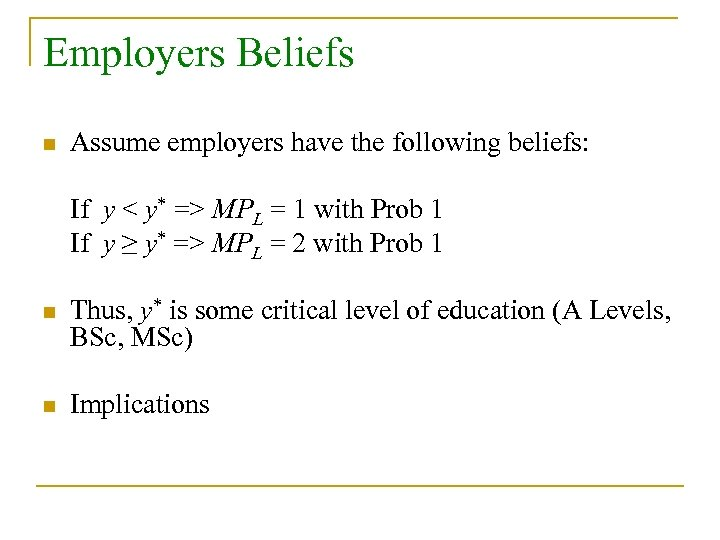Employers Beliefs n Assume employers have the following beliefs: If y < y* => MPL = 1 with Prob 1 If y ≥ y* => MPL = 2 with Prob 1 n Thus, y* is some critical level of education (A Levels, BSc, MSc) n Implications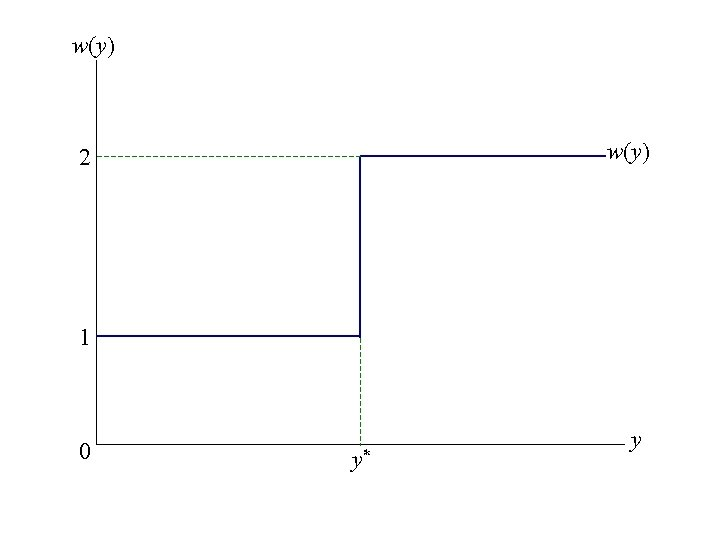w(y) 2 1 0 y* y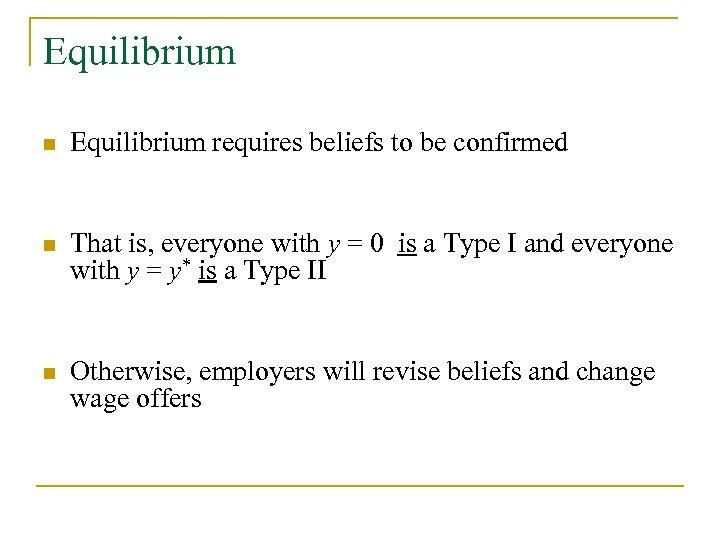Equilibrium n Equilibrium requires beliefs to be confirmed n That is, everyone with y = 0 is a Type I and everyone with y = y* is a Type II n Otherwise, employers will revise beliefs and change wage offers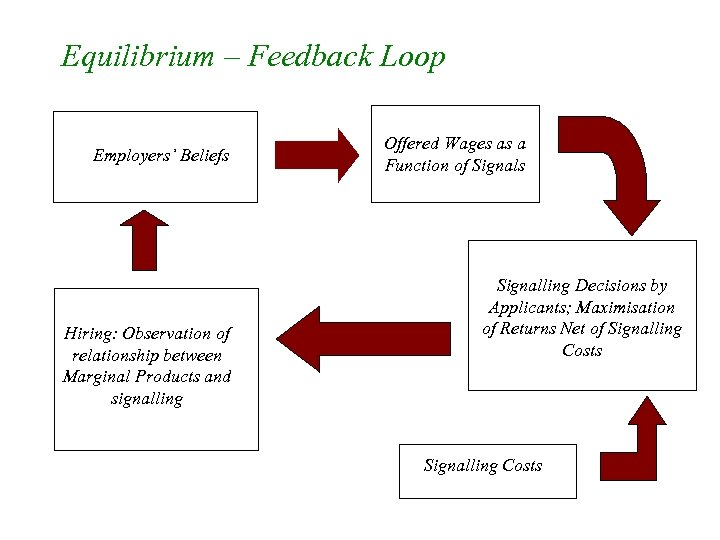Equilibrium – Feedback Loop Employers’ Beliefs Hiring: Observation of relationship between Marginal Products and signalling Offered Wages as a Function of Signals Signalling Decisions by Applicants; Maximisation of Returns Net of Signalling Costs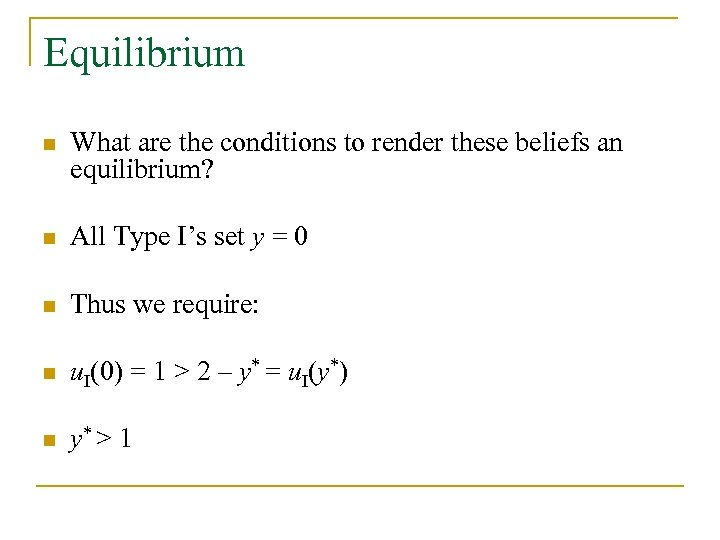Equilibrium n What are the conditions to render these beliefs an equilibrium? n All Type I’s set y = 0 n Thus we require: n u. I(0) = 1 > 2 – y* = u. I(y*) n y* > 1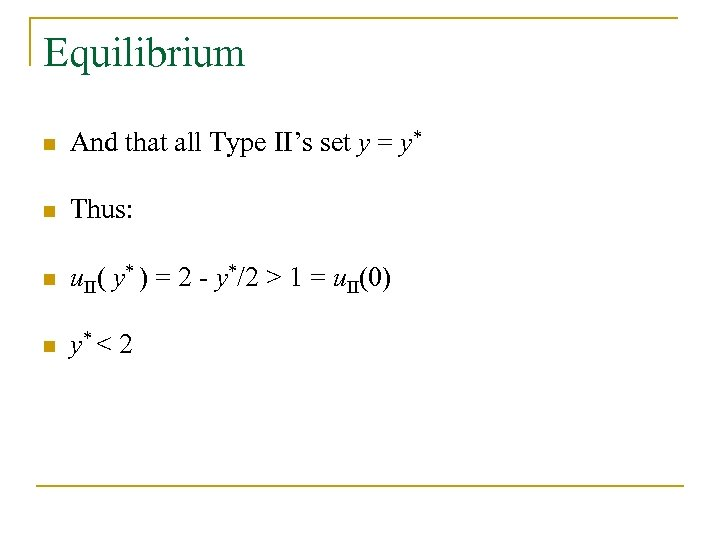Equilibrium n And that all Type II’s set y = y* n Thus: n u. II( y* ) = 2 - y*/2 > 1 = u. II(0) n y* < 2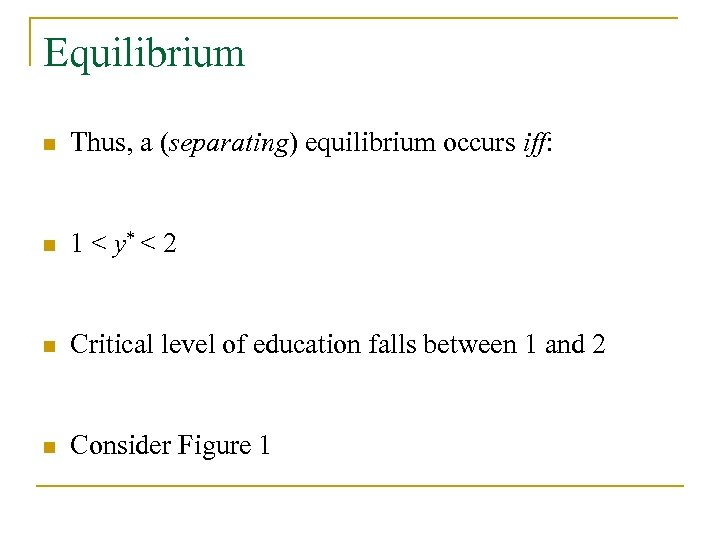Equilibrium n Thus, a (separating) equilibrium occurs iff: n 1 < y* < 2 n Critical level of education falls between 1 and 2 n Consider Figure 1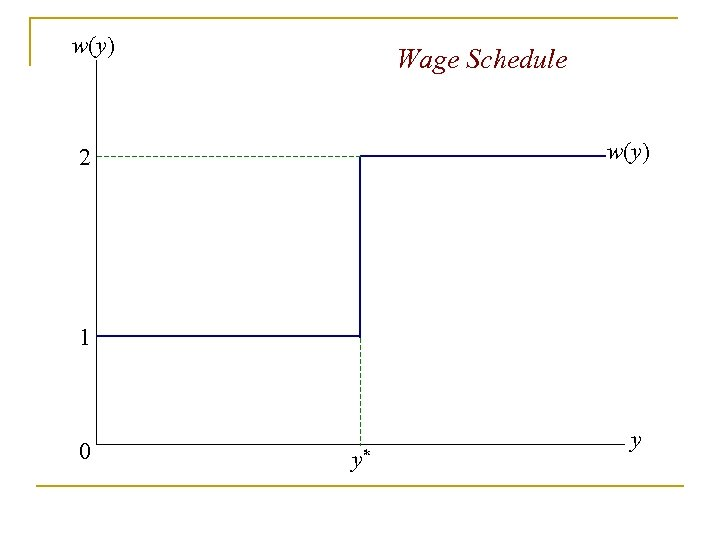w(y) Wage Schedule w(y) 2 1 0 y* y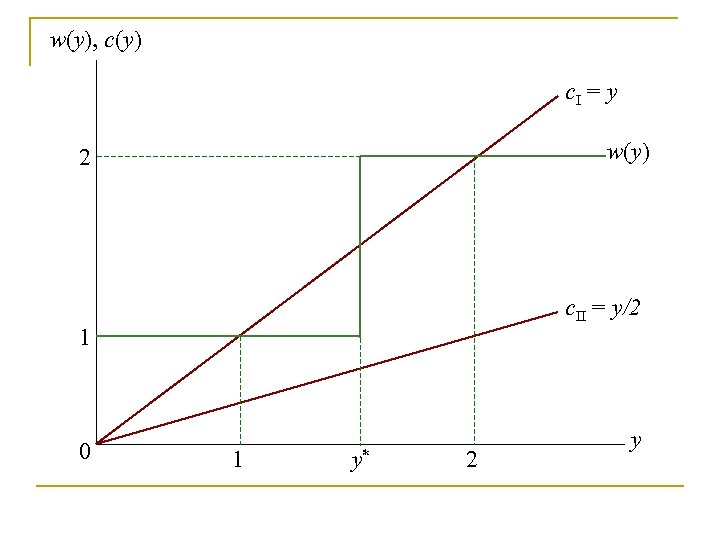w(y), c(y) c. I = y w(y) 2 c. II = y/2 1 0 1 y* 2 yw(y), c(y) c. I = y w(y) 2 c. II = y/2 1 0 1 y* 2 y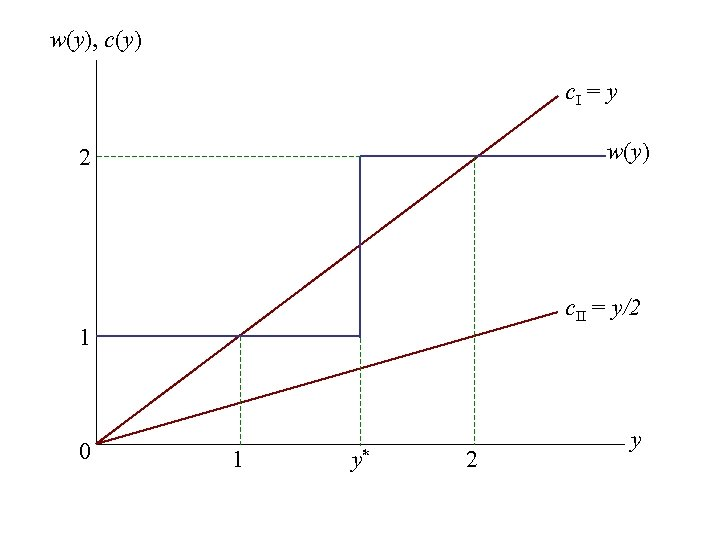w(y), c(y) c. I = y w(y) 2 c. II = y/2 1 0 1 y* 2 y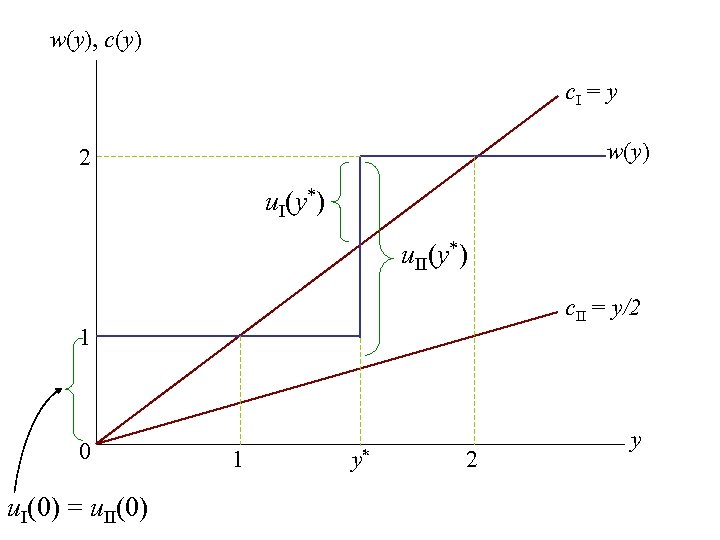w(y), c(y) c. I = y w(y) 2 u. I(y*) u. II(y*) c. II = y/2 1 0 u. I(0) = u. II(0) 1 y* 2 y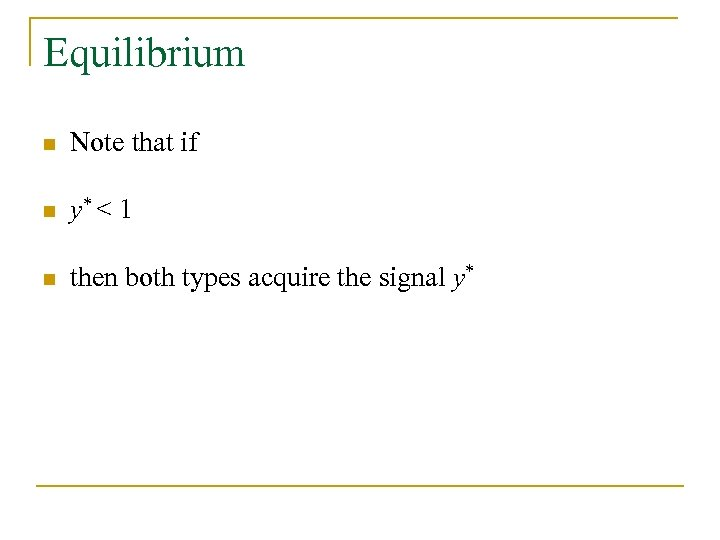Equilibrium n Note that if n y* < 1 n then both types acquire the signal y*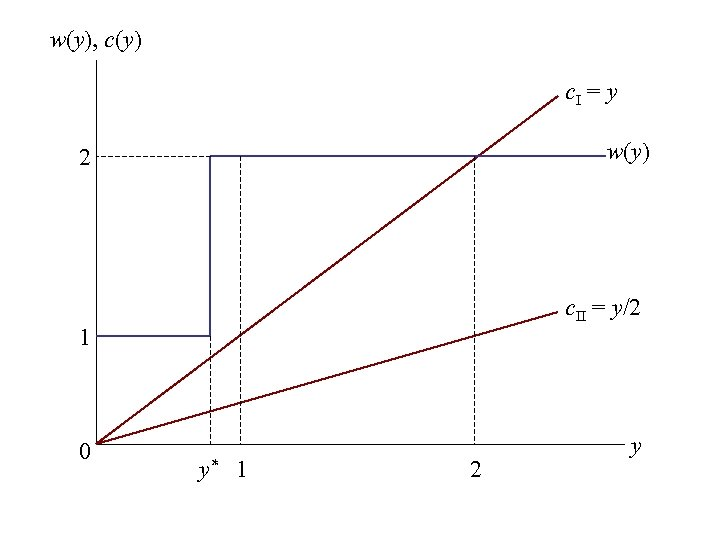w(y), c(y) c. I = y w(y) 2 c. II = y/2 1 0 y* 1 2 y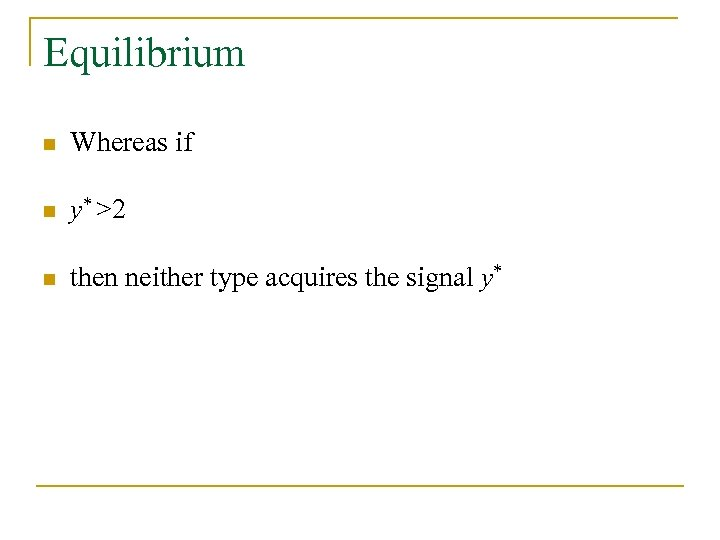Equilibrium n Whereas if n y* >2 n then neither type acquires the signal y*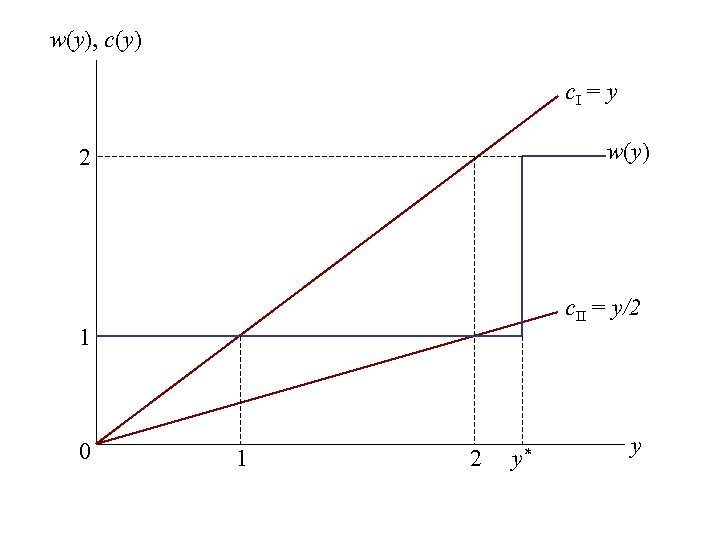w(y), c(y) c. I = y w(y) 2 c. II = y/2 1 0 1 2 y* y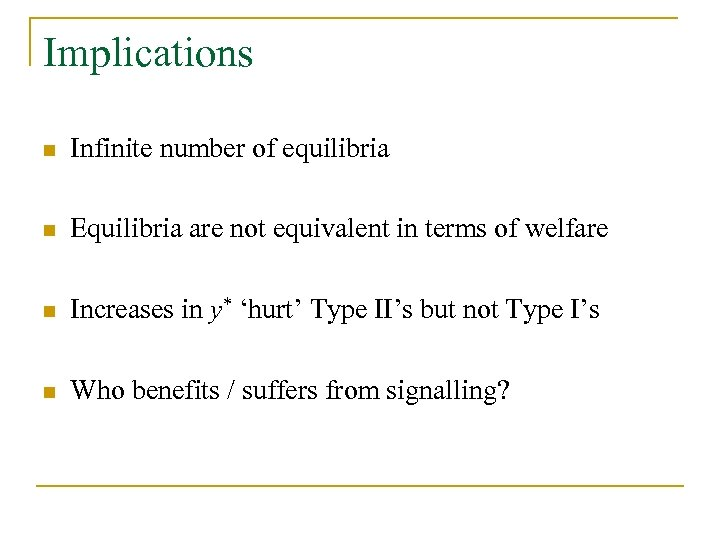Implications n Infinite number of equilibria n Equilibria are not equivalent in terms of welfare n Increases in y* ‘hurt’ Type II’s but not Type I’s n Who benefits / suffers from signalling?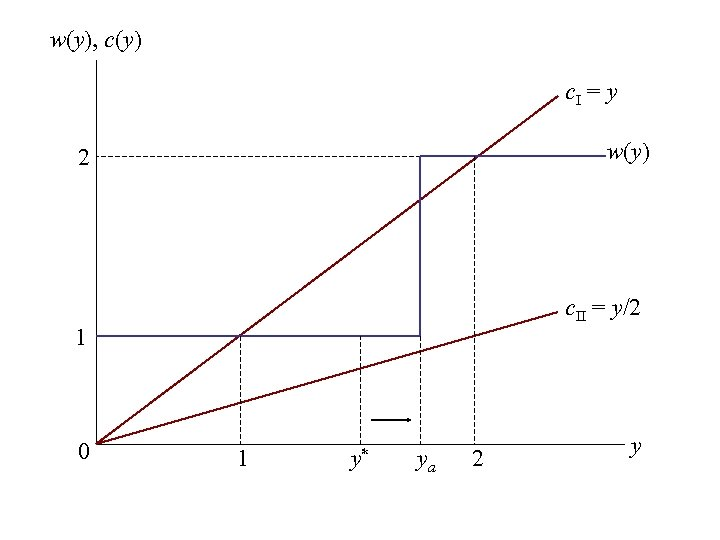w(y), c(y) c. I = y w(y) 2 c. II = y/2 1 0 1 y* ya 2 y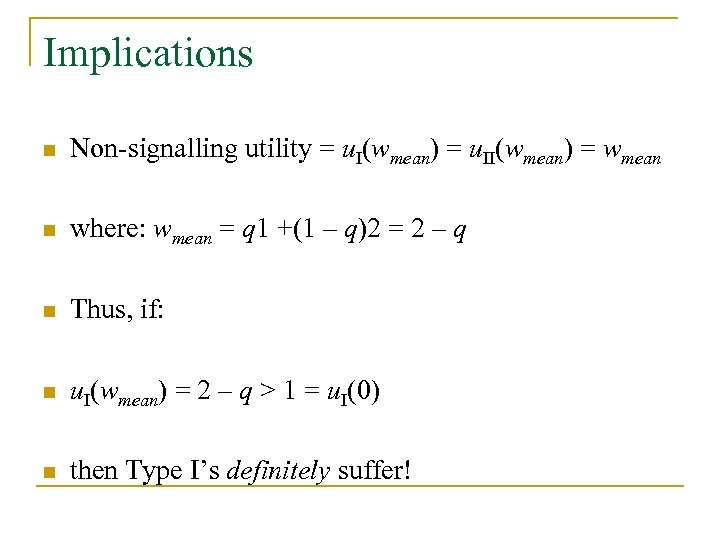Implications n Non-signalling utility = u. I(wmean) = u. II(wmean) = wmean n where: wmean = q 1 +(1 – q)2 = 2 – q n Thus, if: n u. I(wmean) = 2 – q > 1 = u. I(0) n then Type I’s definitely suffer!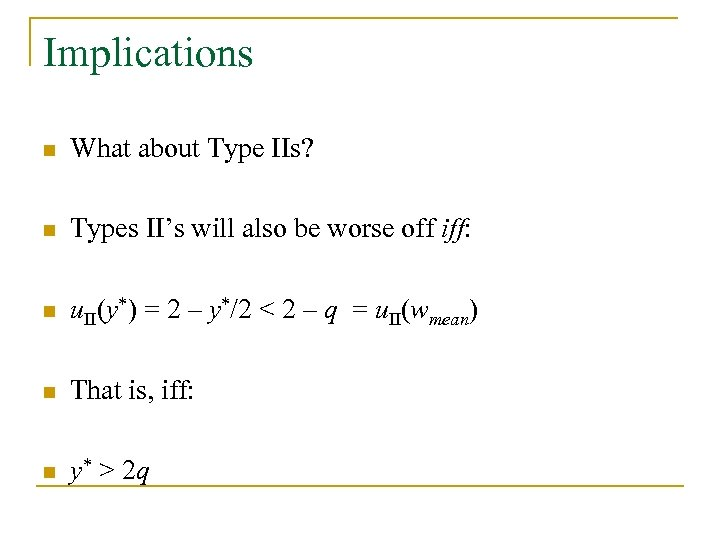Implications n What about Type IIs? n Types II’s will also be worse off iff: n u. II(y*) = 2 – y*/2 < 2 – q = u. II(wmean) n That is, iff: n y* > 2 q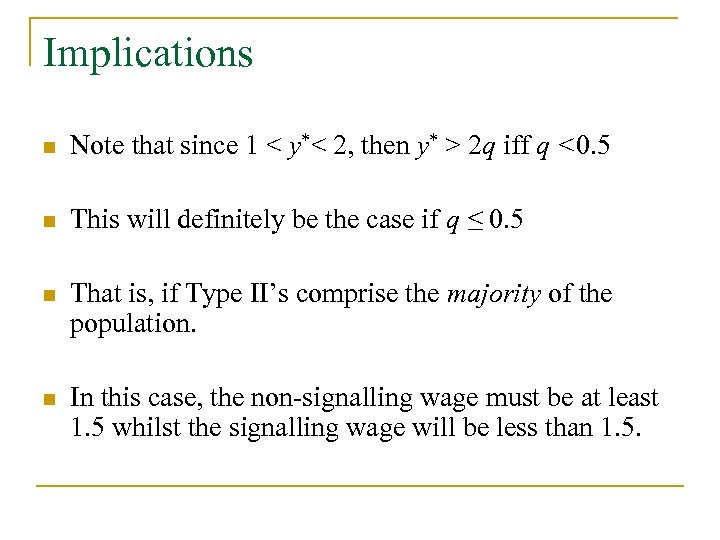Implications n Note that since 1 < y*< 2, then y* > 2 q iff q <0. 5 n This will definitely be the case if q ≤ 0. 5 n That is, if Type II’s comprise the majority of the population. n In this case, the non-signalling wage must be at least 1. 5 whilst the signalling wage will be less than 1. 5.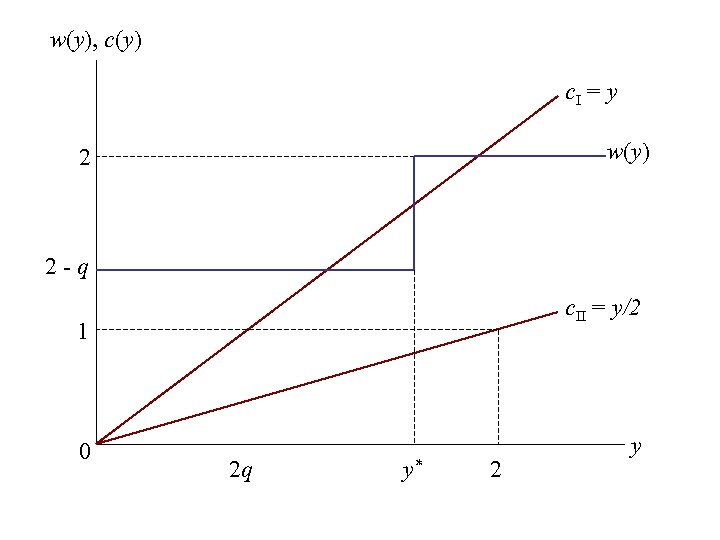w(y), c(y) c. I = y w(y) 2 2 -q c. II = y/2 1 0 2 q y* 2 y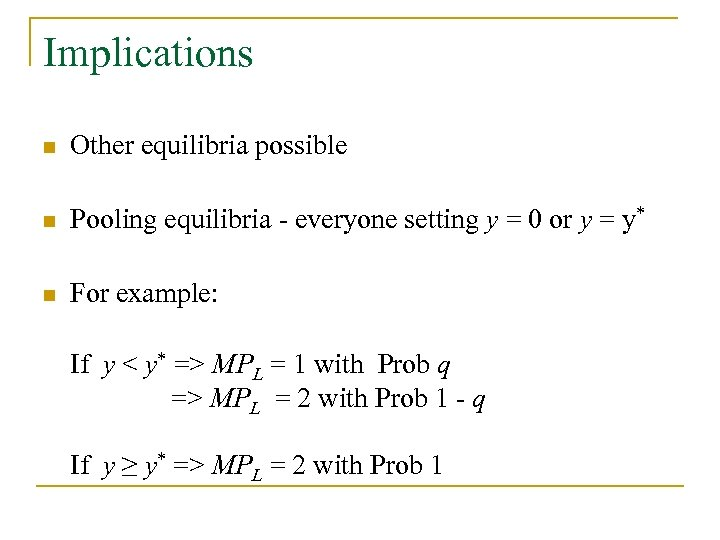Implications n Other equilibria possible n Pooling equilibria - everyone setting y = 0 or y = y* n For example: If y < y* => MPL = 1 with Prob q If y < y* => MPL = 2 with Prob 1 - q If y ≥ y* => MPL = 2 with Prob 1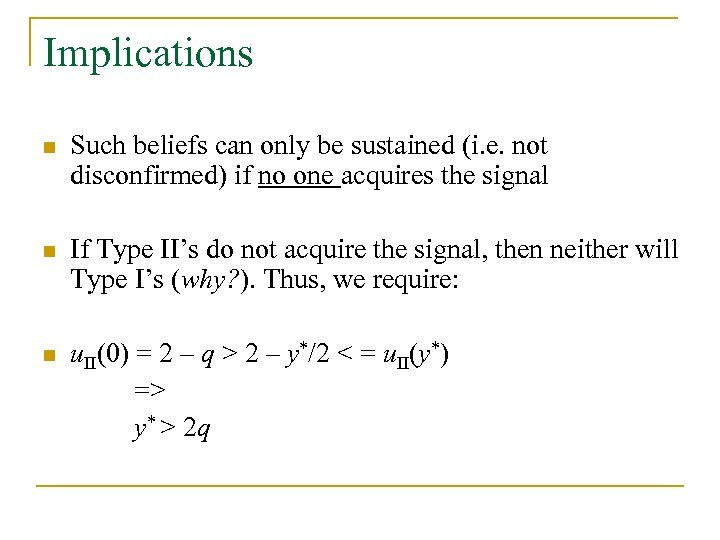Implications n Such beliefs can only be sustained (i. e. not disconfirmed) if no one acquires the signal n If Type II’s do not acquire the signal, then neither will Type I’s (why? ). Thus, we require: n u. II(0) = 2 – q > 2 – y*/2 < = u. II(y*) => y* > 2 q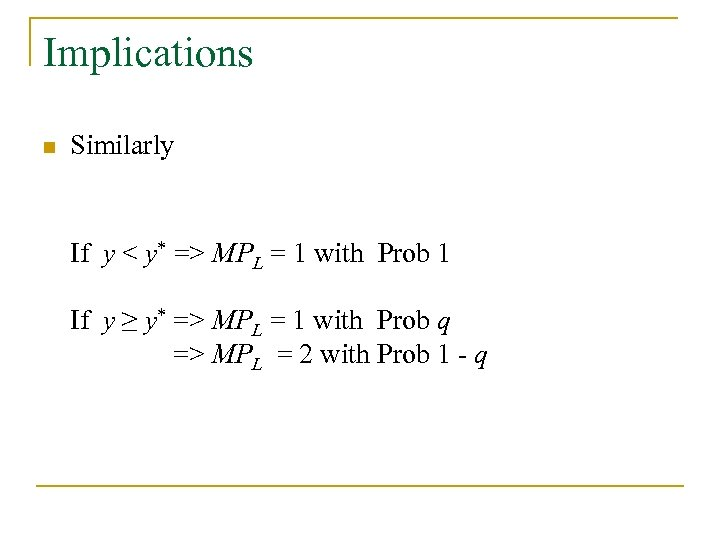Implications n Similarly If If y < y* => MPL = 1 with Prob 1 y MPL = 1 with Prob q y ≥ y* => MPL = 2 with Prob 1 - q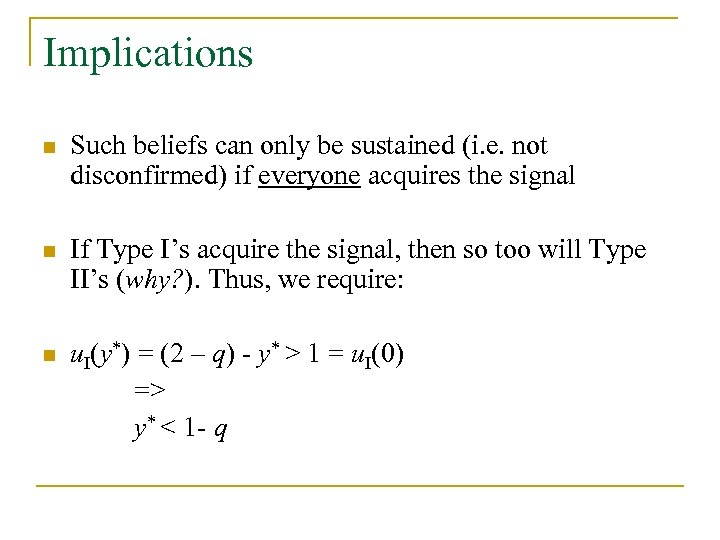Implications n Such beliefs can only be sustained (i. e. not disconfirmed) if everyone acquires the signal n If Type I’s acquire the signal, then so too will Type II’s (why? ). Thus, we require: n u. I(y*) = (2 – q) - y* > 1 = u. I(0) => y* < 1 - q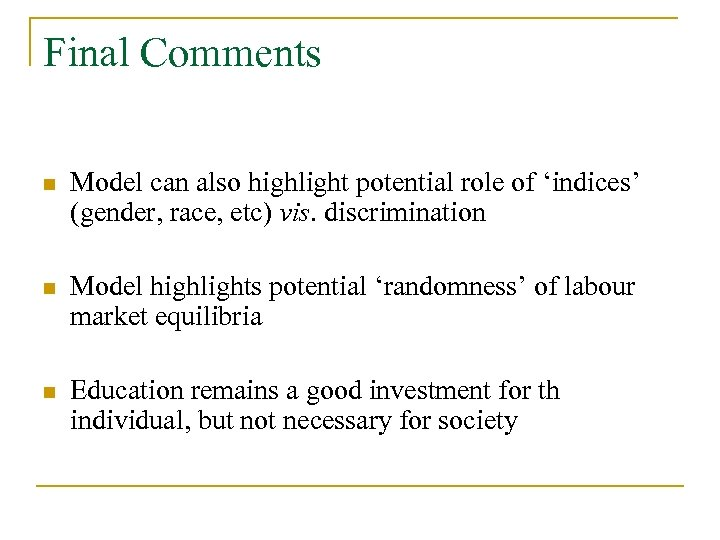Final Comments n Model can also highlight potential role of ‘indices’ (gender, race, etc) vis. discrimination n Model highlights potential ‘randomness’ of labour market equilibria n Education remains a good investment for th individual, but not necessary for society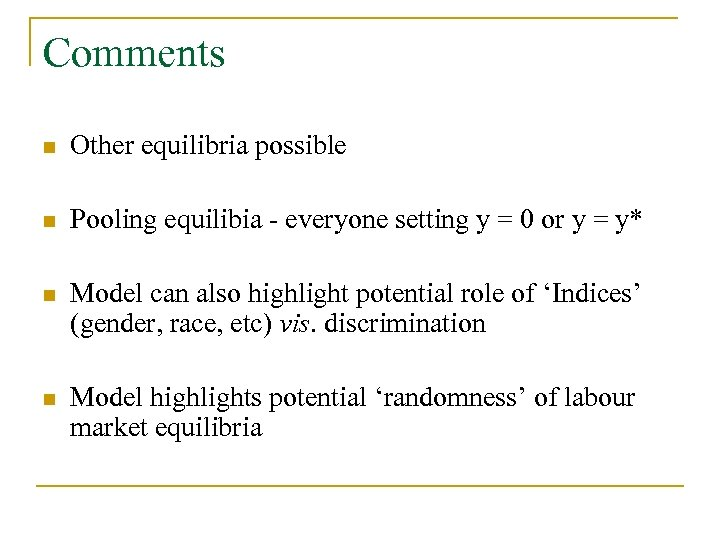Comments n Other equilibria possible n Pooling equilibia - everyone setting y = 0 or y = y* n Model can also highlight potential role of ‘Indices’ (gender, race, etc) vis. discrimination n Model highlights potential ‘randomness’ of labour market equilibria